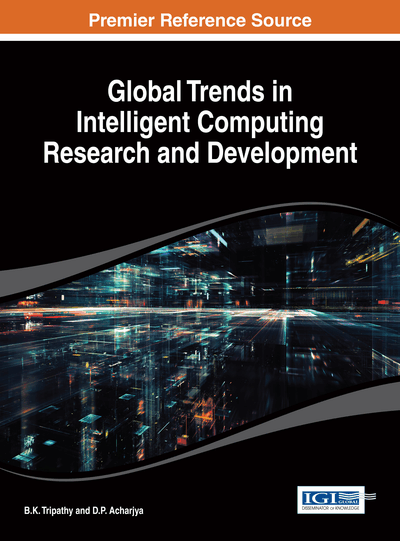# Rough Sets and Approximate Reasoning

B. K. Tripathy (VIT University, India)
DOI: 10.4018/978-1-4666-4936-1.ch008

## Abstract

Several models have been introduced to capture impreciseness in data. Fuzzy sets introduced by Zadeh and Rough sets introduced by Pawlak are two of the most popular such models. In addition, the notion of intuitionistic fuzzy sets introduced by Atanassov and the hybrid models obtained thereof have been very fruitful from the application point of view. The introduction of fuzzy logic and the approximate reasoning obtained through it are more realistic as they are closer to human reasoning. Equality of sets in crisp mathematics is too restricted from the application point of view. Therefore, extending these concepts, three types of approximate equalities were introduced by Novotny and Pawlak using rough sets. These notions were found to be restrictive in the sense that they again boil down to equality of sets and also the lower approximate equality is artificial. Keeping these points in view, three other types of approximate equalities were introduced by Tripathy in several papers. These approximate equalities were further generalised to cover the approximate equalities of fuzzy sets and intuitionistic fuzzy sets by him. In addition, considering the generalisations of basic rough sets like the covering-based rough sets and multigranular rough sets, the study has been carried out further. In this chapter, the authors provide a comprehensive study of all these forms of approximate equalities and illustrate their applicability through several examples. In addition, they provide some problems for future work.
Chapter Preview
Top

## Introduction

Almost all concepts we use in natural languages are vague. So, the theories based on crisp notions like the crisp set theory (Set theory based upon Cantor’s notion of sets (Cantor, 1983) have low utility in real life situations. Uncertainty is usually associated with the boundary region approach, which is based upon the observation that existence of objects which cannot be uniquely classified relative to a set or its complement. This was first formulated in 1893 by the father of modern logic, German logician, Gottlob Frege (Dummet, 1967). Vagueness is not allowed in classical mathematics based on set theory; interesting for philosophy and is a nettlesome problem for natural language, cognitive science, artificial intelligence, machine learning, philosophy and computer science. The exploit of the tolerance for imprecision, uncertainty and partial truth is essential to achieve tractability, robustness and low solution cost.

This has led to the development of several imprecise models extending the crisp set model so far. One of the foremost of such models is the notion of fuzzy sets introduced by L.A.Zadeh (Zadeh, 1965) in 1965. Fuzzy sets capture impreciseness in data through the notion of graded membership of elements instead of the binary membership defined through the characteristic functions for crisp sets. Later on this basic model has been extended to several other general models like the notion of intuitionistic fuzzy sets by K.T.Attanasov (Atanassov, 1986) in 1982 and the notion of interval valued fuzzy sets. Approximate reasoning uses fuzzy sets and fuzzy logic to model human reasoning. It lacks the precision of the exact reasoning in classical logic but it is more effective dealing with complex and ill-defined systems.

Another such important model is the notion of rough sets introduced by (Pawlak, 1982). In the beginning it was supposed to be a competing model to fuzzy sets. But, later on it was shown in (Dubois and Prade, 1990) in 1990 that far from being competitive these models complement each other and they introduced the hybrid models by combining these two models. Imprecision in this approach is expressed by the boundary region of a set. In fact, the idea of rough set is based upon approximation of a set by a pair of sets, called the lower and upper approximation of the set.

Comparison of sets plays a major role in classical set theory. Two sets are said to be equal in crisp set theory iff they have the same elements. This notion is independent of the user or more precisely the user knowledge about the universe dealt with. When we move to the representation of approximate knowledge through rough sets the usual comparisons lose their meaning and in a sense or of no use. To bring about more meaning into such comparisons of rough sets which translate into approximate comparison of knowledge bases,(Novotny and Pawlak, 1985a,1985b,1985c) introduced three notions of rough equalities (bottom, top and total) in 1985 and established several of their properties. This is an important feature from the application point of view. The reason being that, in certain cases it might not be possible for us to conclude about the equality of two sets from the available knowledge in the mathematical sense. But, we can only say that, according to our state of knowledge, they have close features which are enough to assume that they are approximately equal. That is, basing upon our knowledge and requirement we can assume that the two sets are indistinguishable. These concepts are used to achieve approximate reasoning using rough sets which is in the same lines as approximate reasoning using fuzzy sets and fuzzy logic.

It was observed by (Tripathy, Mitra and Ojha, 2008) that even these notions of approximate equalities are restricted in the sense that they boil down to equality of sets. So, the notions of rough equivalences were introduced and were found to be more realistic. Carrying out his study further (Tripathy, 2011) introduced two other types of approximate equalities, namely approximate rough equalities and approximate rough equivalences. A comparative analysis of these four sets of approximate equalities has shown that the notion of approximate rough equivalence is the best and most applicable in real life situations.

## Complete Chapter List

Search this Book:
Reset